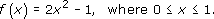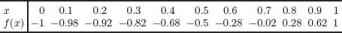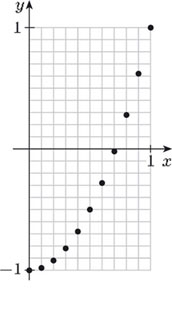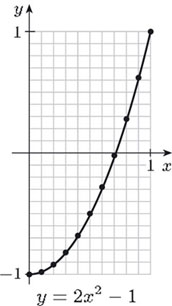Real functions and graphs

This free course is available to start right now. Review the full course description and key learning outcomes and create an account and enrol if you want a free statement of participation.

Free course

1 Overview

A fundamental concept in mathematics is that of a function.

Consider, for example, the function f defined byThis is an example of a real function, because it associates with a given real number x the real number 2x2 − 1: it maps real numbers to real numbers.

One way of picturing this function is the following. First, draw up a table of values, listing in the first row several values of x, and in the second row the corresponding values of f(x); for example, f(0.8) = 2(0.8)2 − 1 = 0.28.Each column in the table is essentially an ordered pair of the form (x, f(x)), which can be plotted as a unique point in the plane. We refer to the set of all such points as the graph of f.Of course, it is not possible to plot all the points of the graph for a function like f, which is defined for infinitely many values of x. Fortunately, for many graphs plotting a few points provides a good idea of what the graph looks like. For any so-called ‘well-behaved’ function, these points can be joined up by a smooth curve – and extended if necessary – to complete the picture.No doubt you are already familiar with this method of drawing a graph. However, it is not always the most efficient method to use when knowledge about the key features of the graph is all that is wanted. For the purposes of this course, that is all that is usually required.

M208_4